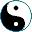Examples of Natural Transformations
<<
>>
 Remember point functors? Now, if in category C we have an arrow f: a → b, this arrow defines a natural transformation from Pointa to Pointb. There is a one-to-one match between transformations of Point functors and arrows of a category. Let's take two sets, A, B, and a function f: A → B. This function determines a natural tranformation between functors (- ⨯A),(- ⨯B): Set → Set.   (- ⨯f): (- ⨯A) → (- ⨯B) by the following formula: (x,a) ↦ (x,f(a)). I feel a temptation to write the definition like this: ```(define (cartesian f) (lambda (x a) (list x (f a))))``` Since for every set A there is a function A → (.), where (.) is a singleton set, we have a natural transformation (- ⨯A) → 1 that for each object X is just a projection: X ⨯ A → X.